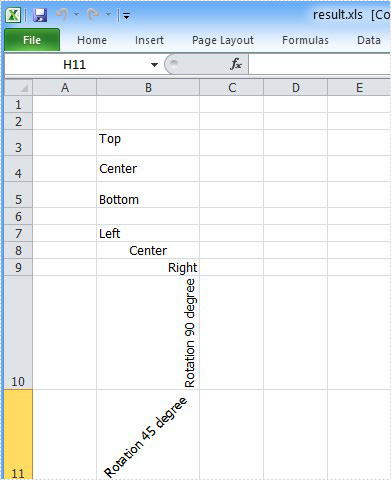# How to Align Excel Text in C#

Spire.XLS specially designed for programmers enables developers to input, edit and delete the text in Excel file. It also enables developers to align text in Excel.

In this article, a solution is introduced to show how to align text in Excel file. Set the vertical alignment using the property VerticalAlignment. Set the horizontal alignment using the property HorizontalAlignment. And set the rotation degree using the property Rotation.

Step 1: Set the vertical alignment

Set the vertical alignment of "B3" to Top.

[C#]
```sheet.Range["B3"].Text = "Top";
sheet.Range["B3"].Style.VerticalAlignment = VerticalAlignType.Top;
```

Set the vertical alignment of "B4" to Center.

[C#]
```sheet.Range["B4"].Text = "Center";
sheet.Range["B4"].Style.VerticalAlignment = VerticalAlignType.Center;
```

Set the vertical alignment of "B5" to Bottom.

[C#]
```sheet.Range["B5"].Text = "Bottom";
sheet.Range["B5"].Style.VerticalAlignment = VerticalAlignType.Bottom;
```

Step 2: Set the horizontal alignment

Set the horizontal alignment of "B7" to Left.

[C#]
```sheet.Range["B7"].Text = "Left";
sheet.Range["B7"].Style.HorizontalAlignment = HorizontalAlignType.Left;
```

Set the horizontal alignment of "B8" to Center.

[C#]
```sheet.Range["B8"].Text = "Center";
sheet.Range["B8"].Style.HorizontalAlignment = HorizontalAlignType.Center;
```

Set the horizontal alignment of "B9" to Right.

[C#]
```sheet.Range["B9"].Text = "Right";
sheet.Range["B9"].Style.HorizontalAlignment = HorizontalAlignType.Right;
```

Step 3: Set the rotation degree

Set the rotation degree of "B10" to 90(counter-clockwise direction).

[C#]
```sheet.Range["B10"].Text = "Rotation 90 degree";
sheet.Range["B10"].Style.Rotation = 90;
```

Set the rotation degree of "B11" to 45(counter-clockwise direction).

[C#]
```sheet.Range["B11"].Text = "Rotation 45 degree";
sheet.Range["B11"].Style.Rotation = 45;
```

Full code and screenshot:```using Spire.Xls;
namespace AlignExcelText
{
class Program
{

static void Main(string[] args)
{
Workbook workbook = new Workbook();
Worksheet sheet = workbook.Worksheets;

sheet.Range["B3"].Text = "Top";
sheet.Range["B3"].Style.VerticalAlignment = VerticalAlignType.Top;

sheet.Range["B4"].Text = "Center";
sheet.Range["B4"].Style.VerticalAlignment = VerticalAlignType.Center;

sheet.Range["B5"].Text = "Bottom";
sheet.Range["B5"].Style.VerticalAlignment = VerticalAlignType.Bottom;

sheet.Range["B7"].Text = "Left";
sheet.Range["B7"].Style.HorizontalAlignment = HorizontalAlignType.Left;

sheet.Range["B8"].Text = "Center";
sheet.Range["B8"].Style.HorizontalAlignment = HorizontalAlignType.Center;

sheet.Range["B9"].Text = "Right";
sheet.Range["B9"].Style.HorizontalAlignment = HorizontalAlignType.Right;

sheet.Range["B10"].Text = "Rotation 90 degree";
sheet.Range["B10"].Style.Rotation = 90;

sheet.Range["B11"].Text = "Rotation 45 degree";
sheet.Range["B11"].Style.Rotation = 45;

sheet.AllocatedRange.AutoFitColumns();
sheet.Range["B3:B5"].RowHeight = 20;

workbook.SaveToFile("result.xls",ExcelVersion.Version97to2003);
}

}
}
```